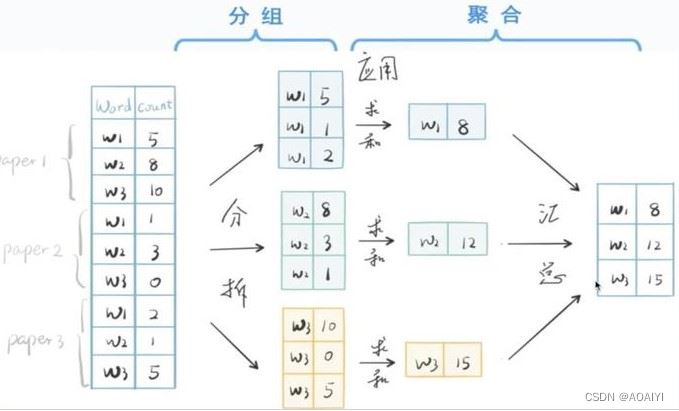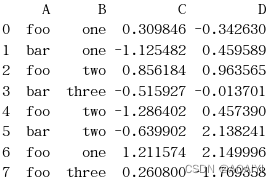# pandas中groupby操作實現

## 二、實驗原理

groupby(by=None, axis=0, level=None, as_index=True, sort=True, group_keys=True, squeeze=False）

• by是指分組依據（列表、字典、函數，元組，Series）
• axis：是作用維度（0為行，1為列）
• level：根據索引級別分組
• sort：對groupby分組後新的dataframe中索引進行排序，sort=True為升序，
• as_index：在groupby中使用的鍵是否成為新的dataframe中的索引，默認as_index=True
• group_keys：在調用apply時，將group鍵添加到索引中以識別片段
• squeeze ：如果可能的話，減少返回類型的維數，否則返回一個一致的類型

grouping操作（split-apply-combine）

pandas中的groupby提供瞭一個高效的數據的分組運算。

1.拆分數據（split）

2.應用某個函數（apply）

3.匯總計算結果（aggregate）Step1 ：數據分組—— groupby 方法

Step2 ：數據聚合：

Python 3.6.1

Jupyter

## 五、實驗步驟

1.創建一個數據幀df。

```import numpy as np
import pandas as pd
df = pd.DataFrame({'A' : ['foo', 'bar', 'foo', 'bar', 'foo', 'bar', 'foo', 'foo'],'B' : ['one', 'one', 'two', 'three', 'two', 'two', 'one', 'three'],'C' : np.random.randn(8),'D' : np.random.randn(8)})
print(df)
```2.通過A列對df進行分佈操作。

`df.groupby('A') `3.通過A、B列對df進行分組操作。

`df.groupby(['A','B']) `4…使用自定義函數進行分組操作，自定義一個函數，使用groupby方法並使用自定義函數給定的條件，按列對df進行分組。

```def get_letter_type(letter):
if letter.lower() in 'aeiou':
return 'vowel'
else:
return 'consonant'

grouped = df.groupby(get_letter_type, axis=1)
for group in grouped:
print(group)
```5.創建一個Series名為s，使用groupby根據s的索引對s進行分組，返回分組後的新Series，對新Series進行first、last、sum操作。

```lst = [1, 2, 3, 1, 2, 3]
s = pd.Series([1, 2, 3, 10, 20, 30], lst)
grouped = s.groupby(level=0)
#查看分組後的第一行數據
grouped.first()
#查看分組後的最後一行數據
grouped.last()
#對分組的各組進行求和
grouped.sum()
```6.分組排序，使用groupby進行分組時，默認是按分組後索引進行升序排列，在groupby方法中加入sort=False參數，可以進行降序排列。

```df2=pd.DataFrame({'X':['B','B','A','A'],'Y':[1,2,3,4]})
#按X列對df2進行分組，並求每組的和
df2.groupby(['X']).sum()
#按X列對df2進行分組，分組時不對鍵進行排序，並求每組的和
df2.groupby(['X'],sort=False).sum()
```7.使用get_group方法得到分組後某組的值。

```df3 = pd.DataFrame({'X' : ['A', 'B', 'A', 'B'], 'Y' : [1, 4, 3, 2]})
#按X列df3進行分組，並得到A組的df3值
df3.groupby(['X']).get_group('A')
#按X列df3進行分組，並得到B組的df3值
df3.groupby(['X']).get_group('B')
```8.使用groups方法得到分組後所有組的值。

```df.groupby('A').groups
df.groupby(['A','B']).groups
```9.多級索引分組，創建一個有兩級索引的Series，並使用兩個方法對Series進行分組並求和。

```arrays = [['bar', 'bar', 'baz', 'baz', 'foo', 'foo', 'qux', 'qux'],['one', 'two', 'one', 'two', 'one', 'two', 'one', 'two']]
index=pd.MultiIndex.from_arrays(arrays,names=['first','second'])
s=pd.Series(np.random.randn(8),index=index)
s.groupby(level=0).sum()
s.groupby(level='second').sum()
```10.復合分組，對s按first、second進行分組並求和。

```s.groupby(level=['first', 'second']).sum()
```11.復合分組（按索引和列），創建數據幀df，使用索引級別和列對df進行分組。

```arrays = [['bar', 'bar', 'baz', 'baz', 'foo', 'foo', 'qux', 'qux'],['one', 'two', 'one', 'two', 'one', 'two', 'one', 'two']]
index = pd.MultiIndex.from_arrays(arrays, names=['first', 'second'])
df = pd.DataFrame({'A': [1, 1, 1, 1, 2, 2, 3, 3], 'B': np.arange(8)},index=index)
print(df)
df.groupby([pd.Grouper(level=1),'A']).sum()
```12.對df進行分組，將分組後C列的值賦值給grouped，統計grouped中每類的個數。

```df = pd.DataFrame({'A' : ['foo', 'bar', 'foo', 'bar', 'foo', 'bar', 'foo', 'foo'],'B' : ['one', 'one', 'two', 'three', 'two', 'two', 'one', 'three'],'C' : np.random.randn(8),'D' : np.random.randn(8)})
grouped=df.groupby(['A'])
grouped_C=grouped['C']
print(grouped_C.count())
```13.對上面創建的df的C列，按A列值進行分組並求和。

`df['C'].groupby(df['A']).sum() `14.遍歷分組結果，通過A，B兩列對df進行分組，分組結果的組名為元組。

```for name, group in df.groupby(['A', 'B']):
print(name)
print(group)
```15.通過A列對df進行分組，並查看分組對象的bar列。

`df.groupby(['A']).get_group(('bar')) `16.按A,B兩列對df進行分組，並查看分組對象中bar、one都存在的部分。

`df.groupby(['A','B']).get_group(('bar','one')) `17.聚合操作，按A列對df進行分組，使用聚合函數aggregate求每組的和。

`grouped=df.groupby(['A']) grouped.aggregate(np.sum) ````grouped=df.groupby(['A'])
grouped.aggregate(np.sum)
```18.當as_index=True時，在groupby中使用的鍵將成為新的dataframe中的索引。按A、B兩列對df進行分組，這是使參數as_index=False，再使用聚合函數aggregate求每組的和.

```grouped=df.groupby(['A','B'],as_index=False)
grouped.aggregate(np.sum)
```19.聚合操作，按A、B列對df進行分組，使用size方法，求每組的大小。返回一個Series，索引是組名，值是每組的大小。

```grouped=df.groupby(['A','B'])
grouped.size()
```20.聚合操作，對分組grouped進行統計描述。

`grouped.describe() `